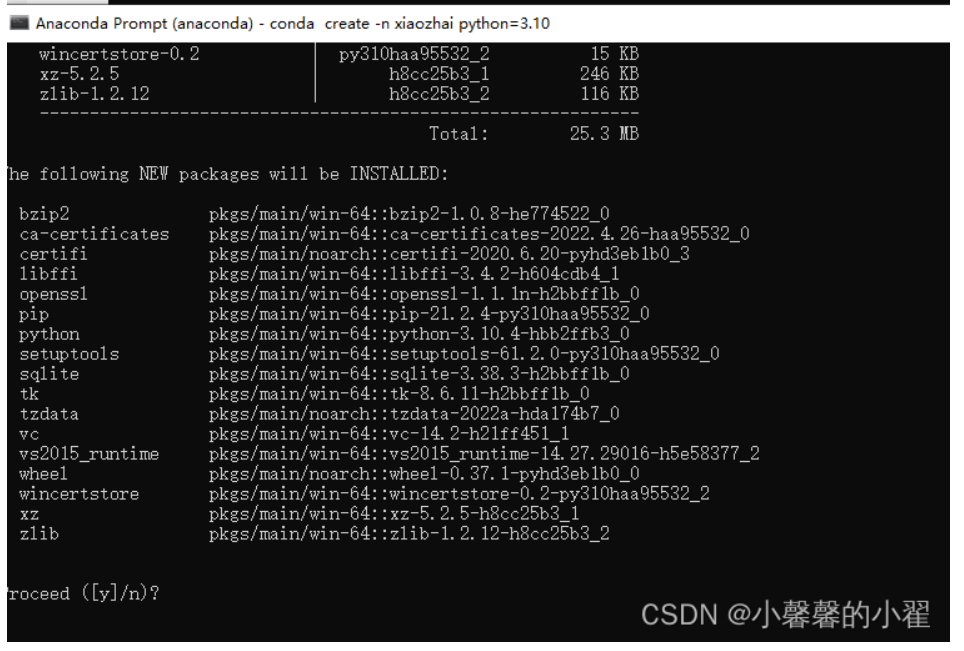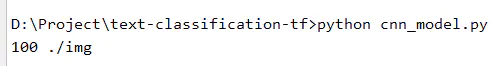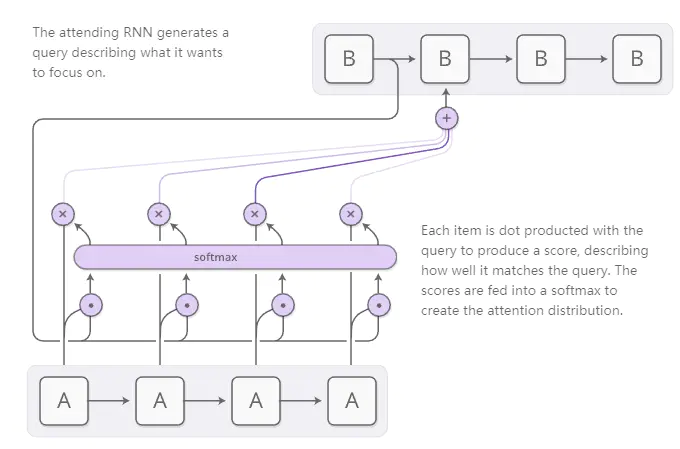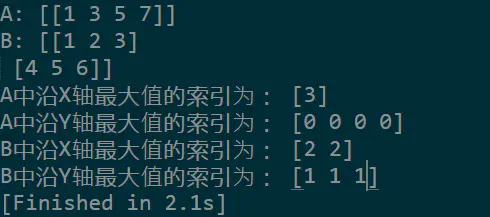+关注继续查看

# Control Flow

Note: Functions taking Tensor arguments can also take anything accepted by tf.convert_to_tensor.

## Control Flow Operations

TensorFlow provides several operations and classes that you can use to control the execution of operations and add conditional dependencies to your graph.

### tf.identity(input, name=None)

Return a tensor with the same shape and contents as the input tensor or value.

##### Args:
• input: A Tensor.
• name: A name for the operation (optional).
##### Returns:

Tensor. Has the same type as input.

### tf.tuple(tensors, name=None, control_inputs=None)

Group tensors together.

This creates a tuple of tensors with the same values as the tensors argument, except that the value of each tensor is only returned after the values of all tensors have been computed.

control_inputs contains additional ops that have to finish before this op finishes, but whose outputs are not returned.

This can be used as a "join" mechanism for parallel computations: all the argument tensors can be computed in parallel, but the values of any tensor returned by tuple are only available after all the parallel computations are done.

See also group and with_dependencies.

##### Args:
• tensors: A list of Tensors or IndexedSlices, some entries can be None.
• name: (optional) A name to use as a name_scope for the operation.
• control_inputs: List of additional ops to finish before returning.
##### Returns:

Same as tensors.

##### Raises:
• ValueError: If tensors does not contain any Tensor or IndexedSlices.

### tf.group(*inputs, **kwargs)

Create an op that groups multiple operations.

When this op finishes, all ops in input have finished. This op has no output.

See also tuple and with_dependencies.

##### Args:
• *inputs: One or more tensors to group.
• **kwargs: Optional parameters to pass when constructing the NodeDef.
• name: A name for this operation (optional).
##### Returns:

An Operation that executes all its inputs.

##### Raises:
• ValueError: If an unknown keyword argument is provided, or if there are
       no inputs.


### tf.no_op(name=None)

Does nothing. Only useful as a placeholder for control edges.

##### Args:
• name: A name for the operation (optional).
##### Returns:

The created Operation.

### tf.count_up_to(ref, limit, name=None)

Increments 'ref' until it reaches 'limit'.

This operation outputs "ref" after the update is done. This makes it easier to chain operations that need to use the updated value.

##### Args:
• ref: A mutable Tensor. Must be one of the following types: int32int64. Should be from a scalar Variable node.
• limit: An int. If incrementing ref would bring it above limit, instead generates an 'OutOfRange' error.
• name: A name for the operation (optional).
##### Returns:

Tensor. Has the same type as ref. A copy of the input before increment. If nothing else modifies the input, the values produced will all be distinct.

## Logical Operators

TensorFlow provides several operations that you can use to add logical operators to your graph.

### tf.logical_and(x, y, name=None)

Returns the truth value of x AND y element-wise.

##### Args:
• x: A Tensor of type bool.
• y: A Tensor of type bool.
• name: A name for the operation (optional).
##### Returns:

Tensor of type bool.

### tf.logical_not(x, name=None)

Returns the truth value of NOT x element-wise.

##### Args:
• x: A Tensor of type bool.
• name: A name for the operation (optional).
##### Returns:

Tensor of type bool.

### tf.logical_or(x, y, name=None)

Returns the truth value of x OR y element-wise.

##### Args:
• x: A Tensor of type bool.
• y: A Tensor of type bool.
• name: A name for the operation (optional).
##### Returns:

Tensor of type bool.

### tf.logical_xor(x, y, name='LogicalXor')

x ^ y = (x | y) & ~(x & y).

## Comparison Operators

TensorFlow provides several operations that you can use to add comparison operators to your graph.

### tf.equal(x, y, name=None)

Returns the truth value of (x == y) element-wise.

##### Args:
• x: A Tensor. Must be one of the following types: float32float64int32int64complex64quint8qint8qint32.
• y: A Tensor. Must have the same type as x.
• name: A name for the operation (optional).
##### Returns:

Tensor of type bool.

### tf.not_equal(x, y, name=None)

Returns the truth value of (x != y) element-wise.

##### Args:
• x: A Tensor. Must be one of the following types: float32float64int32int64complex64quint8qint8qint32.
• y: A Tensor. Must have the same type as x.
• name: A name for the operation (optional).
##### Returns:

Tensor of type bool.

### tf.less(x, y, name=None)

Returns the truth value of (x < y) element-wise.

##### Args:
• x: A Tensor. Must be one of the following types: float32float64int32int64.
• y: A Tensor. Must have the same type as x.
• name: A name for the operation (optional).
##### Returns:

Tensor of type bool.

### tf.less_equal(x, y, name=None)

Returns the truth value of (x <= y) element-wise.

##### Args:
• x: A Tensor. Must be one of the following types: float32float64int32int64.
• y: A Tensor. Must have the same type as x.
• name: A name for the operation (optional).
##### Returns:

Tensor of type bool.

### tf.greater(x, y, name=None)

Returns the truth value of (x > y) element-wise.

##### Args:
• x: A Tensor. Must be one of the following types: float32float64int32int64.
• y: A Tensor. Must have the same type as x.
• name: A name for the operation (optional).
##### Returns:

Tensor of type bool.

### tf.greater_equal(x, y, name=None)

Returns the truth value of (x >= y) element-wise.

##### Args:
• x: A Tensor. Must be one of the following types: float32float64int32int64.
• y: A Tensor. Must have the same type as x.
• name: A name for the operation (optional).
##### Returns:

Tensor of type bool.

### tf.select(condition, t, e, name=None)

Selects elements from t or e, depending on condition.

The conditiont, and e tensors must all have the same shape, and the output will also have that shape. The condition tensor acts as an element-wise mask that chooses, based on the value at each element, whether the corresponding element in the output should be taken from t (if true) or e (if false). For example:

For example:

# 'condition' tensor is [[True, False]
#                        [True, False]]
# 't' is [[1, 1],
#         [1, 1]]
# 'e' is [[2, 2],
#         [2, 2]]
select(condition, t, e) ==> [[1, 2],
[1, 2]]

##### Args:
• condition: A Tensor of type bool.
• t: A Tensor with the same shape as condition.
• e: A Tensor with the same type and shape as t.
• name: A name for the operation (optional).
##### Returns:

Tensor with the same type and shape as t and e.

### tf.where(input, name=None)

Returns locations of true values in a boolean tensor.

This operation returns the coordinates of true elements in input. The coordinates are returned in a 2-D tensor where the first dimension (rows) represents the number of true elements, and the second dimension (columns) represents the coordinates of the true elements. Keep in mind, the shape of the output tensor can vary depending on how many true values there are in input. Indices are output in row-major order.

For example:

# 'input' tensor is [[True, False]
#                    [True, False]]
# 'input' has two true values, so output has two coordinates.
# 'input' has rank of 2, so coordinates have two indices.
where(input) ==> [[0, 0],
[1, 0]]

# input tensor is [[[True, False]
#                     [True, False]]
#                    [[False, True]
#                     [False, True]]
#                    [[False, False]
#                     [False, True]]]
# 'input' has 5 true values, so output has 5 coordinates.
# 'input' has rank of 3, so coordinates have three indices.
where(input) ==> [[0, 0, 0],
[0, 1, 0],
[1, 0, 1],
[1, 1, 1],
[2, 1, 1]]

##### Args:
• input: A Tensor of type bool.
• name: A name for the operation (optional).
##### Returns:

Tensor of type int64.

## Debugging Operations

TensorFlow provides several operations that you can use to validate values and debug your graph.

### tf.is_finite(x, name=None)

Returns which elements of x are finite.

##### Args:
• x: A Tensor. Must be one of the following types: float32float64.
• name: A name for the operation (optional).
##### Returns:

Tensor of type bool.

### tf.is_inf(x, name=None)

Returns which elements of x are Inf.

##### Args:
• x: A Tensor. Must be one of the following types: float32float64.
• name: A name for the operation (optional).
##### Returns:

Tensor of type bool.

### tf.is_nan(x, name=None)

Returns which elements of x are NaN.

##### Args:
• x: A Tensor. Must be one of the following types: float32float64.
• name: A name for the operation (optional).
##### Returns:

Tensor of type bool.

### tf.verify_tensor_all_finite(t, msg, name=None)

Assert that the tensor does not contain any NaN's or Inf's.

##### Args:
• t: Tensor to check.
• msg: Message to log on failure.
• name: A name for this operation (optional).
##### Returns:

Same tensor as t.

### tf.check_numerics(tensor, message, name=None)

Checks a tensor for NaN and Inf values.

When run, reports an InvalidArgument error if tensor has any values that are not a number (NaN) or infinity (Inf). Otherwise, passes tensor as-is.

##### Args:
• tensor: A Tensor. Must be one of the following types: float32float64.
• message: A string. Prefix of the error message.
• name: A name for the operation (optional).
##### Returns:

Tensor. Has the same type as tensor.

### tf.add_check_numerics_ops()

Connect a check_numerics to every floating point tensor.

check_numerics operations themselves are added for each float or double tensor in the graph. For all ops in the graph, the check_numerics op for all of its (float or double) inputs is guaranteed to run before the check_numerics op on any of its outputs.

##### Returns:

group op depending on all check_numerics ops added.

### tf.Assert(condition, data, summarize=None, name=None)

Asserts that the given condition is true.

If condition evaluates to false, print the list of tensors in datasummarize determines how many entries of the tensors to print.

##### Args:
• condition: The condition to evaluate.
• data: The tensors to print out when condition is false.
• summarize: Print this many entries of each tensor.
• name: A name for this operation (optional).

### tf.Print(input_, data, message=None, first_n=None, summarize=None, name=None)

Prints a list of tensors.

This is an identity op with the side effect of printing data when evaluating.

##### Args:
• input_: A tensor passed through this op.
• data: A list of tensors to print out when op is evaluated.
• message: A string, prefix of the error message.
• first_n: Only log first_n number of times. Negative numbers log always;
    this is the default.

• summarize: Only print this many entries of each tensor.
• name: A name for the operation (optional).
##### Returns:

Same tensor as input_.0 00 00 00 0Tensorflow教程(7) 命令行参数tf.flags的使用
Tensorflow教程(7) 命令行参数tf.flags的使用
0 0TensorFlow教程(6) tf.Variable() 和tf.get_variable()
TensorFlow教程(6) tf.Variable() 和tf.get_variable()
0 0TensorFlow教程(5) 随机数实例
TensorFlow教程(5) 随机数实例
0 0TensorFlow教程(4)-Attention机制
TensorFlow教程(4)-Attention机制
0 0TensorFlow教程(3)-embedding_lookup用法
TensorFlow教程(3)-embedding_lookup用法
0 0TensorFlow教程(2)-基本函数使用

0 0138727911